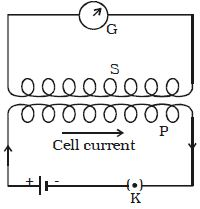# How to work Mutual Induction?

When a steady current flows in one coil as in the left illustration, a magnetic field is produced in the other coil. Suppose, P and S are two coils placed close to each other (Figure). P is connected to a battery through a key K. S is connected to a galvanometer G. On pressing K, current in P starts increasing from zero to a maximum value.As the flow of current increases, the magnetic flux linked with P increases. Therefore, magnetic flux linked with S also increases producing an induced emf in S. Now, the galvanometer shows the deflection. According to Lenz’s law the induced current in S would oppose the increase in current in P by flowing in a direction opposite to the current in P, thus delaying the growth of current to the maximum value. When the key ‘K’ is released, current starts decreasing from maximum to zero value, consequently magnetic flux linked with P decreases. Therefore magnetic flux linked with S also decreases and hence, an emf is induced in S. According to Lenz’s law, the induced current in S flows in such a direction so as to oppose the decrease in current in P thus prolonging the decay of current.